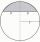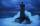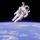Elevation

What must be the elevation of an observer in order that he may be able to see an object on the earth 536 km away? Assume the earth to be a smooth sphere with radius 6378.1 km.

Result

h =  22482.4 m

Solution:Leave us a comment of example and its solution (i.e. if it is still somewhat unclear...):Be the first to comment!To solve this verbal math problem are needed these knowledge from mathematics:

Pythagorean theorem is the base for the right triangle calculator. See also our trigonometric triangle calculator.

Next similar examples:

1. Above EarthTo what height must a boy be raised above the earth in order to see one-fifth of its surface.
2. Sphere and coneWithin the sphere of radius G = 33 cm inscribe cone with largest volume. What is that volume and what are the dimensions of the cone?
3. Billiard ballsA layer of ivory billiard balls of radius 6.35 cm is in the form of a square. The balls are arranged so that each ball is tangent to every one adjacent to it. In the spaces between sets of 4 adjacent balls other balls rest, equal in size to the original. T
4. Sphere cutsAt what distance from the center intersects sphere with radius R = 56 plane, if the cut area and area of the main sphere circle is in ratio 1/2.
5. Spherical capWhat is the surface area of a spherical cap, the base diameter 20 m, height 2 m.
6. Cube and sphereCube with the surface area 150 cm2 is described sphere. What is sphere surface?
7. HorizonThe top of a lighthouse is 17 m above the sea. How far away is an object which is just “on the horizon”? [Assume the earth is a sphere of radius 6378.1 km.]
8. Rotation of the EarthCalculate the circumferential speed of the Earth's surface at a latitude of 61°​​. Consider a globe with a radius of 6378 km.
9. FelixCalculate how much land saw Felix Baumgartner after jump from 32 km above ground. The radius of the Earth is R = 6378 km.
10. MoonWe see Moon in the perspective angle 28'. Moon's radius is 1740 km at the time of the full moon. Calculate the mean distance of the Moon from the Earth.
11. AirplaneAviator sees part of the earth's surface with an area of 200,000 square kilometers. How high he flies?
12. CubesOne cube is inscribed sphere and the other one described. Calculate difference of volumes of cubes, if the difference of surfaces in 257 mm2.
13. Two ballsTwo balls, one 8cm in radius and the other 6cm in radius, are placed in a cylindrical plastic container 10cm in radius. Find the volume of water necessary to cover them.
14. Sphere - partsCalculate the area of a spherical cap, which is part of an area with base radius ρ = 9 cm and a height v = 3.1 cm.
15. Cube in sphereThe sphere is inscribed cube with edge 8 cm. Find the radius of the sphere.
16. Sphere equationObtain the equation of sphere its centre on the line 3x+2z=0=4x-5y and passes through the points (0,-2,-4) and (2,-1,1).
17. Sphere from tree pointsEquation of sphere with three point (a,0,0), (0, a,0), (0,0, a) and center lies on plane x+y+z=a